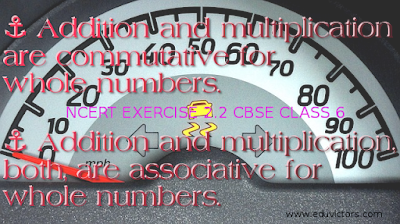## Whole Numbers - NCERT Ex 2.2

CBSE Class 6

Q1. Find the sum by suitable rearrangement:
(a) 837 + 208 + 363
(b) 1962 + 453 + 1538 + 647

(a) 837 + 208 + 363
= (837 + 363) + 208
= 1200 + 208
= 1408

(b) 1962 + 453 + 1538 + 647
= (1962 + 1538) + (453 + 647)
= 3500 + 1100
= 4600

Q2. Find the product by suitable rearrangement:
(a) 2 × 1768 × 50
(b) 4 × 166 × 25
(c) 8 × 291 × 125
(d) 625 × 279 × 16
(e) 285 × 5 × 60
(f) 125 × 40 × 8 × 25

(a) 2 × 1768 × 50
= (2 × 50) × 1768
= 100 × 1768
= 176800

(b) 4 × 166 × 25
=  (4 × 25) × 166
= 100 × 166
= 16600

(c) 8 × 291 × 125
= (8 × 125) × 291
= 1000 × 291
= 291000

(d) 625 × 279 × 16
=  (625 × 16) × 279
= 10000 × 279
= 2790000

(e) 285 × 5 × 60
= 285 × (5 × 60)
= 285 × 300
= 85500

(f) 125 × 40 × 8 × 25
= (125 × 8) × (40 × 25)
= 1000 × 1000
= 10,00,000

Q3. Find the value of the following:
(a) 297 × 17 + 297 × 3
(b) 54279 × 92 + 8 × 54279
(c) 81265 × 169 – 81265 × 69
(d) 3845 × 5 × 782 + 769 × 25 × 218

a) 297 × 17 + 297 × 3
= 297 × (17 + 3)
= 297 × 20
= 5940

(b) 54279 × 92 + 8 × 542379
= 54279 × (92 + 8)
= 54279 × 100
= 5427900

(c) 81265 × 169 – 81265 × 69
= 81265 × (169 – 69)
= 81265 × 100
= 8126500

(d) 3845 × 5 × 782 + 769 × 25 × 218
= 3845 × 5 × 782 + 769 × 5 × 5 × 218)
= 3845 × 5 × 782 + 3845 × 5 × 218
= 3845 × 5 × (782 + 218)
= 3845 × 5 × 1000
= 19225000

Q4. Find the product using suitable properties.
(a) 738 × 103
(b) 854 × 102
(c) 258 × 1008
(d) 1005 × 168

(a) 738 × 103
= 738 × (100 + 3)
= 738 × 100 + 738 × 3
= 73800 + 2214
= 76014

(b) 854 × 102
= 854 × (100 + 2)
= 854 × 100 + 854 × 2
= 85400 + 1708
= 87108

(c) 258 × 1008
= 258 × (1000 + 8)
= 258 × 1000 + 258 × 8
= 258000 + 2064
= 260064

(d) 1005 × 168
= (1000 + 5) × 168
= 1000 × 168 + 5 × 168
= 168000 + 840
= 168840

Q5. A taxidriver filled his car petrol tank with 40 litres of petrol on Monday. The next day, he filled the tank with 50 litres of petrol. If the petrol costs Rs 44 per litre, how much did he spend in all on petrol?

Petrol filled on Monday = 40 litres
Petrol filled on next day = 50 litres
Total petrol filled = 90 litres

Now,Cost of 1 litre petrol = ₹44
Cost of 90 litres petrol = 44 × 90
= 44 × (100 – 10)
= 44 × 100 – 44 × 10
= 4400 – 440 = ₹3960

Thus, he spent ₹ 3960 on petrol.

Q6. A vendor supplies 32 litres of milk to a hotel in the morning and 68 litres of milk in the evening. If the milk costs Rs 15 per litre, how much money is due to the vendor per day?

Supply of milk in morning = 32 litres
Supply of milk in evening = 68 litres

Total supply = 32 + 68 = 100 litres

Now Cost of 1 litre milk = ₹15
Cost of 100 litres milk = 15 × 100 = ₹1500

∴ ₹1500 is due to the vendor per day

Q7. Match the following:
(i) 425 × 136 = 425 × (6 + 30 +100) (a) Commutativity under multiplication.
(ii) 2 × 49 × 50 = 2 × 50 × 49 (b) Commutativity under addition.
(iii) 80 + 2005 + 20 = 80 + 20 + 2005 (c) Distributivity of multiplication over addition.